### Create an Account

Already have account?

### Forgot Your Password ?

Home / Questions / x,y max -(x² + y2) ż (y + 2) sx v 3 s.t. 1.5+y 2 (a) What is the solution of the unconstra...

# x,y max -(x² + y2) ż (y + 2) sx v 3 s.t. 1.5+y 2 (a) What is the solution of the unconstrained problem? Answer: (b) At this part, you should consider each restriction at a time: i. What is the

x,y max -(x² + y2) ż (y + 2) sx v 3 s.t. 1.5+y 2 (a) What is the solution of the unconstrained problem? Answer:
(b) At this part, you should consider each restriction at a time: i. What is the solution of the problem restricted only by Ineqı)? Answer: ii. And only by (Ineq2)? Answer:
(c) What is the solution of the problem when both constraints hold in equality, i.e., x.y max -(x+y?) 3 z (y + 2) = x 1.5+y 2 St. (Eq) = X (E92)
(d) Use the method of slack variables presented in lecture to find the solution for the initial problem. Explain why the solution is different from the one you found in the previous item. Answer:
X. (e) Consider below the modified version of the initial problem: max -(x+y?) 3 s.t. 2 (y + 2) = x 1.5+ y 2x 2 i. What is the solution for this modified problem? Answer: (Ineqı') (Ineq2') ii. What is the role of the constraints now? Answer: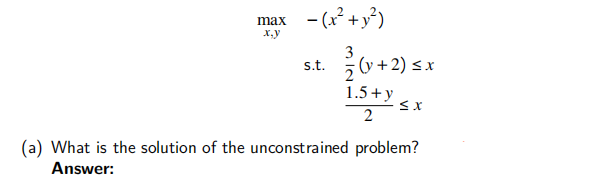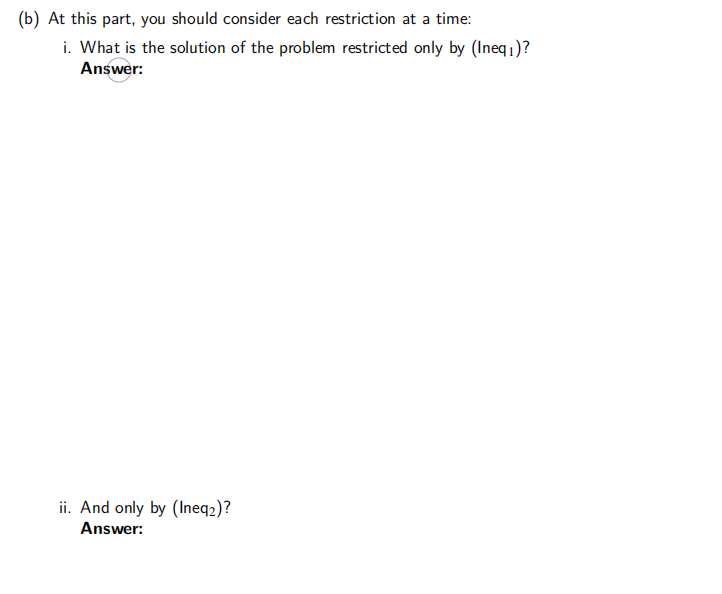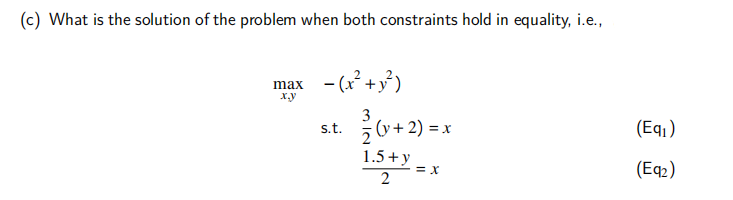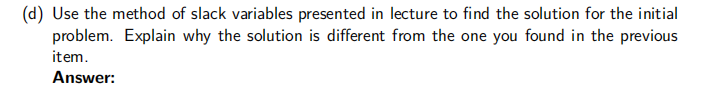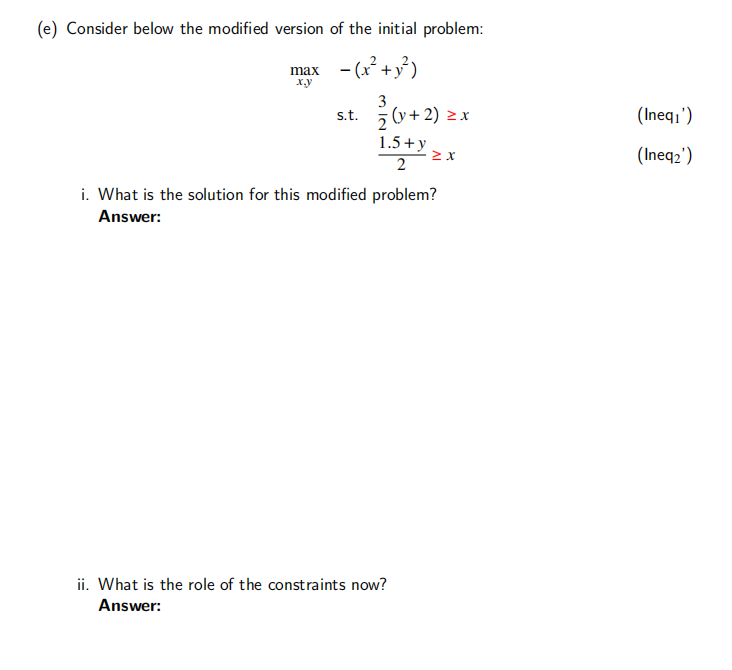Apr 16 2021 View more View Less

#### Answer (Solved)Subscribe To Get Solution